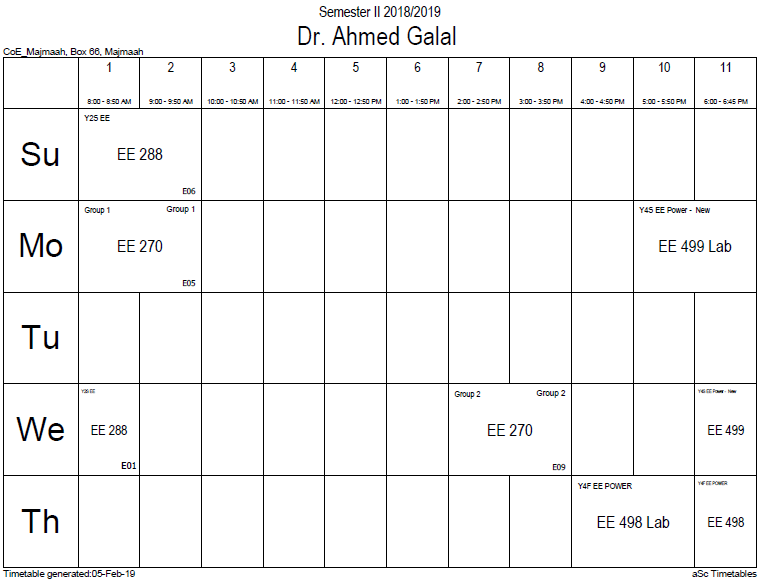## Total harmonic dis

The total harmonic distortion, or THD, of a signal is a measurement of the harmonic distortion present and is defined as the ratio of the sum of the powers of all harmonic components to the power of the fundamental frequency. THD is used to characterize the linearity of audio systems and the power quality of electric power systems.

In audio systems, lower THD means the components in a loudspeaker, amplifier or microphone or other equipment produce a more accurate reproduction by reducing harmonics added by electronics and audio media.

In power systems, lower THD means reduction in peak currents, heating, emissions, and core loss in motors.

## Explanation

To understand a system with an input and an output, such as an audio amplifier, we start with an ideal system where the transfer function is linear and time-invariant. When a signal passes through a non-ideal, non-linear device, additional content is added at the harmonics of the original frequencies. THD is a measurement of the extent of that distortion.

When the input is a pure sine wave, the measurement is most commonly the ratio of the sum of the powers of all higher harmonic frequencies to the power at the first harmonic, or fundamental, frequency:$mbox{THD} = frac{P_2 + P_3 + P_4 + cdots + P_infty}{P_1} = frac{displaystylesum_{i=2}^infty P_i}{P_1}$

which can equivalently be written as$mbox{THD} = frac{P_mathrm{total} - P_1}{P_1}$

if there is no source of power other than the signal and its harmonics.

Measurements based on amplitudes (e.g. voltage or current) must be converted to powers to make addition of harmonics distortion meaningful. For a voltage signal, for example, the ratio of the squares of the RMS voltages is equivalent to the power ratio:$mbox{THD} = frac{V_2^2 + V_3^2 + V_4^2 + cdots + V_infty^2}{V_1^2}$

where Vi is the RMS voltage of ith harmonic and i = 1 is the fundamental frequency.

THD is also commonly defined as an amplitude ratio rather than a power ratio, resulting in a definition of THD which is the square root of that given above:$mbox{THD} = frac{ sqrt{V_2^2 + V_3^2 + V_4^2 + cdots + V_infty^2} }{V_1}$

This latter definition is commonly used in audio distortion (percentage THD) specifications. It is unfortunate that these two conflicting definitions of THD (one as a power ratio and the other as an amplitude ratio) are both in common usage.

As a result, THD is a non-standardized specification and the results between manufacturers are not easily comparable. Since individual harmonic amplitudes are measured, it is required that the manufacturer disclose the test signal frequency range, level and gain conditions, and number of measurements taken. It is possible to measure the full 20–20 kHz range using a sweep. For all signal processing equipment, except microphone preamplifiers, the preferred gain setting is unity. For microphone preamplifiers, standard practice is to use maximum gain.

Measurements for calculating the THD are made at the output of a device under specified conditions. The THD is usually expressed in percent as distortion factor or in dB relative to the fundamental as distortion attenuation.

### Office HoursNo office hours### My Timetable### Contactsemail: [email protected]

Phone: 2570

### Welcome

Welcome To Faculty of Engineering### IEEEhttp://www.ieee.org/

/

### Bookmarkshttp://www.utk.edu/research/

http://science.doe.gov/grants/index.asp

http://www1.eere.energy.gov/vehiclesandfuels/

http://www.eere.energy.gov/

### Upcoming Conferences### Engineering quotes### Travel Web Siteshttp://www.hotels.com/

http://www.orbitz.com/

http://www.hotwire.com/us/index.jsp

http://www.kayak.com/

### Blackboardستقام اختبارات الميدتيرم يوم الثلاثاء 26-6-1440

حسب الجدول المعلن بلوحات الاعلان

### Summer trainingThe registration for summer training will start from 5th week of second semesterClass registration week 1

### برنامج التجسير### إحصائية الموقع

عدد الصفحات: 2879

البحوث والمحاضرات: 1280

الزيارات: 99591